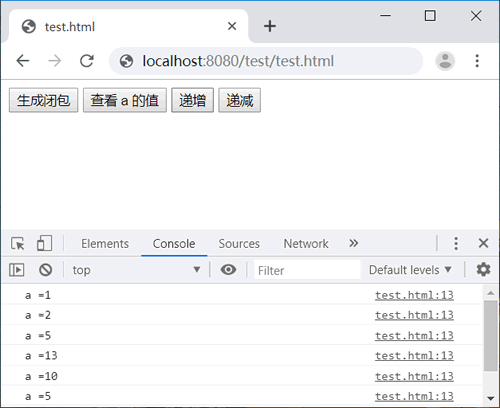• 内容
• 评论
• 相关

## 定义闭包

#### 闭包结构

```function f(x) {  //外部函数
return function (y) {  //内部函数，通过返回内部函数，实现外部引用
return x + y;  //访问外部函数的参数
};
}
var c = f(5);  //调用外部函数，获取引用内部函数
console.log(c(6));  //调用内部函数，原外部函数的参数继续存在```

1. 在 JavaScript 脚本预编译期，声明的函数 f 和变量 c，先被词法预解析。
2. 在 JavaScript 执行期，调用函数 f，并传入值 5。
3. 在解析函数 f 时，将创建执行环境（函数作用域）和活动对象，并把参数和私有变量、内部函数都映射为活动对象的属性。
4. 参数 x 的值为 5，映射到活动对象的 x 属性。
5. 内部函数通过作用域链引用了参数 x，但是还没有被执行。
6. 外部函数被调用后，返回内部函数，导致内部函数被外界变量 c 引用。
7. JavaScript 解析器检测到外部函数的活动对象的属性被外界引用，无法注销该活动对象，于是在内存中继续维持该对象的存在。
8. 当调用 c，即调用内部函数时，可以看到外部函数的参数 x 存储的值继续存在。这样就可以实现后续运算操作，返回 x+y=5=6=11。

```var c;  //声明全局变量
function f(x) {  //外部函数
c = function (y) {  //内部函数，通过向全局变量开放实现外部引用
return x + y;  //访问外部函数的参数
};
}
f(5);  //调用外部函数
console.log(c(6));  //使用全局变量c调用内部函数，返回11```

#### 闭包变体

```var add;  //全局变量
function f() {  //外部函数
var a = [1,2,3];  //私有变量，引用型数组
add = function (x) {  //测试函数，对外开放
a = x * x;  //修改私有数组的元素值
}
return a;  //返回私有数组的引用
}
var c = f();
console.log(c);  //读取闭包内数组，返回1
console.log(c);  //读取闭包内数组，返回25
console.log(c);  //读取闭包内数组，返回100```

```function f(x) {  //外部函数
var a = 1;  //私有变量
return a;
}
var c = f(5);
console.log(c);  //仅是一个值，返回1```

## 使用闭包

#### 示例1

```var f = function () {  //外部函数
var a = [];  //私有数组初始化
return function (x) {  //返回内部函数
a.push(x);  //添加元素
return a;  //返回私有数组
};
} ()  //直接调用函数，生成执行环境
var a = f(1);  //添加值
console.log(a);  //返回1
var b = f(2);  //添加值
console.log(b);  //返回1，2```

#### 示例2

```<script>
function f() {
var a = 1;
b = function () {
console.log("a =" + a);
}
c = function () {
a ++;
}
d = function () {
a --;
}
}
</script>
<button onclick="f()">生成闭包</button>
<button onclick="b()">查看 a 的值</button>
<button onclick="c()">递增</button>
<button onclick="d()">递减</button>```0条评论